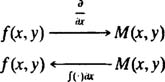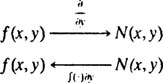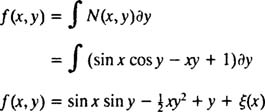## Partial Integration

Suppose it is known that a given function ƒ( x) is the derivative of some function ƒ( x); how is ƒ( x) found? The answer, of course, is to integrate ƒ( x). Now consider a related question: Suppose it is known that a given function ƒ( x, y) is the partial derivative with respect to x of some function F( x, y); how is F( x, y) found? The answer is to integrate ƒ( x, y) with respect to x, a process I refer to as partial integration. Similarly, suppose it is known that a given function ƒ( x, y) is the partial derivative with respect to y of some function ƒ( x, y); how is ƒ( x, y) found? Integrate ƒ( x, y) with respect to y.

Example 1: Let M( x, y) = 2 xy 2 + x 2y. It is known that M equals ƒ x for some function ƒ( x, y). Determine the most general such function ƒ( x, y).

Since M( x, y) is the partial derivative with respect to x of some function ƒ( x, y), M must be partially integrated with respect to x to recover ƒ. This situation can be symbolized as follows:Therefore,Note carefully that the “constant” of integration here is any (differentiable) function of y—denoted by ψ( y)—since any such function would vanish upon partial differentiation with respect to x (just as any pure constant c would vanish upon ordinary differentiation). If the question had asked merely for a function ƒ( x, y) for which ƒ x = M, you could just take ψ( y) ≡ 0.

Example 2: Let N( x, y) = sin x cos yxy + 1. It is known that N equals ƒ y for some function ƒ( x, y). Determine the most general such function ƒ( x, y).

Since N( x, y) is the partial derivative with respect to y of some function ƒ( x, y), N must be partially integrated with respect to y to recover ƒ. This situation can be symbolized as follows:Therefore,Note carefully that the “constant” of integration here is any (differentiable) function of x—denoted by ξ( x)—since any such function would vanish upon partial differentiation with respect to y. If the question had asked merely for a function ƒ( x, y) for which ƒ y = N, you could just take ξ( x) ≡ 0.